Gauge your potential with Aakash BYJU'S JEE Main'23 Mock Test Gauge your potential with Aakash BYJU'S JEE Main'23 Mock Test

# JEE Main 2021 Chemistry Paper With Solutions Feb 24 Shift 2

Students can access the JEE Main 2021 Chemistry question paper that was held on Feb 24 shift 2 along with detailed solutions on this page. Students can go through or start solving the question paper to get familiar with the overall nature of the exam including the question paper pattern, marking scheme, types of question and more. This is a great way to start preparing effectively for the entrance exam.
As students continue to solve the problems and go through the solutions they will be able to understand the topic more clearly and also have a quick revision before the exams. All in all, students will be able to figure out the easiest and quick way to solve any type of questions that may be asked in the exam. Download the JEE Main 2021 Chemistry question paper held on Feb 24 shift 2 instantly from below.

### JEE Main 2021 24th February Chemistry Question Paper

SECTION A

Question 1. The correct order of the following compounds showing increasing tendency towards nucleophilic substitution reaction is:1. a. (iv) < (i) < (iii) < (ii)
2. b. (iv) < (i) < (ii) < (iii)
3. c. (i) < (ii) < (iii) < (iv)
4. d. (iv) < (iii) < (ii) < (i)

Solution:Reactivity ∝ – M group present at o/p position.

Question 2. Match List-I with List-II

List- I List-II

(Metal) (Ores)

(a) Aluminum (i) Siderite

(b) Iron (ii) Calamine

(c) Copper (iii) Kaolinite

(d) Zinc (iv) Malachite

1. a. (a)-(iv), (b)-(iii), (c)-(ii), (d)-(i)
2. b. (a)-(i), (b)-(ii), (c)-(iii), (d)-(iv)
3. c. (a)-(iii), (b)-(i), (c)-(iv), (d)-(ii)
4. d. (a)-(ii), (b)-(iv), (c)-(i), (d)-(iii)

Solution:

Siderite FeCO3

Calamine ZnCO3

Kaolinite Si2Al2O5(OH)4 or Al2O3.2SiO2.2H2O

Malachite CuCO3.Cu(OH)2

Question 3. Match List-I with List-II

List- I List-II

(Salt) (Flame colour wavelength)

(a) LiCl (i) 455.5 nm

(b) NaCl (ii) 970.8 nm

(c) RbCl (iii) 780.0 nm

(d) CsCl (iv) 589.2 nm

Choose the correct Answer from the options given below:

1. a. (a)-(ii), (b)-(i), (c)-(iv), (d)-(iii)
2. b. (a)-(ii), (b)-(iv), (c)-(iii), (d)-(i)
3. c. (a)-(iv), (b)-(ii), (c)-(iii), (d)-(i)
4. d. (a)-(i), (b)-(iv), (c)-(ii), (d)-(iii)

Solution:

Range of visible region: 390 nm – 760 nm

VIBGYOR

Violet - Red

LiCl Crimson Red

NaCl Golden yellow

RbCl Violet

CsCl Blue

So, LiCl which is crimson have wavelengths close to red in the spectrum of visible region which is as per given data.

Question 4. Given below are two statements: one is labelled as Assertion A and the other is labelled as Reason R.

Assertion A: Hydrogen is the most abundant element in the Universe, but it is not the most abundant gas in the troposphere.

Reason R: Hydrogen is the lightest element.

In the light of the above statements, choose the correct Answer from the given below

(1) A is false but R is true

(2) Both A and R are true and R is the correct explanation of A

(3) A is true but R is false

(4) Both A and R are true but R is NOT the correct explanation of A

1. a. A is false but R is true
2. b. Both A and R are true and R is the correct explanation of A
3. c. A is true but R is false
4. d. Both A and R are true but R is NOT the correct explanation of A

Solution:

Hydrogen is the most abundant element in the universe because all luminous bodies of the universe i.e. stars and nebulae are made up of hydrogen which acts as nuclear fuel and fusion reaction is responsible for their light.

Question 5. Given below are two statements:

1. a. Both Statement I and Statement II are false
2. b. Statement I is false but Statement II is true
3. c. Statement I is true but Statement II is false
4. d. Both Statement I and Statement II are true

Solution:

For survival of aquatic life dissolved oxygen is responsible for its optimum limit 6.5 ppm and optimum limit of BOD ranges from 10-20 ppm & BOD stands for biochemical oxygen demand.

Question 6. Which one of the following carbonyl compounds cannot be prepared by addition of water on an alkyne in the presence of HgSO4 and H2SO4?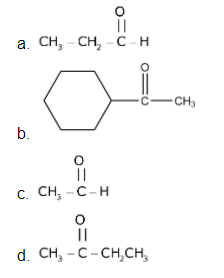Solution:

Reaction of Alkyne with HgSO4 & H2SO4 follow asHence, by this process preparation of CH3CH2CHO can't be possible.

Question 7. Which one of the following compounds is non-aromatic?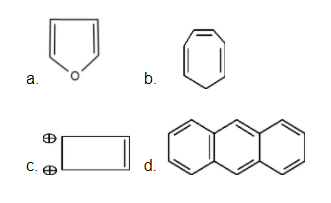Solution:(Not planar)

Hence, it is non-aromatic.

Question 8. The incorrect statement among the following is:

1. a. VOSO4 is a reducing agent
2. b. Red color of ruby is due to the presence of CO3+
3. c. Cr2O3 is an amphoteric oxide
4. d. RuO4 is an oxidizing agent

Solution:

Red color of ruby is due to presence of CrO3 or Cr+6 not CO3+

Question 9. According to Bohr's atomic theory:

(a) Kinetic energy of electron is ∝ Z2 / n2

(b) The product of velocity (v) of electron and principal quantum number (n). ‘vn’ ∝ z2

(c) Frequency of revolution of electron in an orbit is ∝ Z3 / n3

(d) Coulombic force of attraction on the electron is ∝ Z3 / n4

Choose the most appropriate Answer from the options given below

1. a. (c) only
2. b. (a) and (d) only
3. c. (a) only
4. d. (a), (c) and (d) only

Solution:

(a) KE = –TE = 13.6 × Z2 / n2 eV

KE α Z2 / n2

(b) V = 2.188 × 106 × z / n m/s

So, Vn ∝ Z

Frequency = V / 2πr

F α Z2 / n2 [∴ r α Z2 / n2 and v α Z / n ]

(d) Force ∝ Z2 / r2

So, F ∝ Z3 / n4

So, only statement (A) is correct.

Question 10. Match List-I with List-II

List- I List-II

(a) Valium (iv) Tranquilizer

(b) Morphine (iii) Analgesic

(c) Norethindrone (i) Antifertility drug

(d) Vitamin B12 (ii) Pernicious anemia

1. a. (a)-(iv), (b)-(iii), (c)-(ii), (d)-(i)
2. b. (a)-(i), (b)-(iii), (c)-(iv), (d)-(ii)
3. c. (a)-(ii), (b)-(iv), (c)-(iii), (d)-(i)
4. d. (a)-(iv), (b)-(iii), (c)-(i), (d)-(ii)

Solution:

(a) Valium (iv) Tranquilizer

(b) Morphine (iii) Analgesic

(c) Norethindrone (i) Antifertility drug

(d) Vitamin B12 (ii) Pernicious anemia

Question 11. The Correct set from the following in which both pairs are in correct order of melting point is

1. a. LiF > LiCl ; NaCl > MgO
2. b. LiF > LiCl ; MgO > NaCl
3. c. LiCl > LiF ; NaCl > MgO
4. d. LiCl > LiF ; MgO > NaCl

Solution:

Generally,

M.P. ∝ Lattice energy =

$$\frac{KQ_{1}Q_{2}}{r^{+} + r^{-}}$$

∝ (packing efficiency)

Question 12. The calculated magnetic moments (spin only value) for species

[FeCl4]2-, [Co(C2O4)3]3- and MnO2-4 respectively are:

Solution:

[FeCl4]2-

Fe2+ 3d6 → 4 unpaired electrons. as Cl in a weak field liquid.

Question 13. Which of the following reagent is suitable for the preparation of the product in the following reaction?1. a. Red P + Cl2
2. b. NH2-NH2/ C2H5O-Na+
3. c. Ni/H2
4. d. NaBH4

Solution:It is a wolf-kishner reduction of carbonyl compounds.

Question 14. The diazonium salt of which of the following compounds will form a coloured dye on reaction with β-Naphthol in NaOH?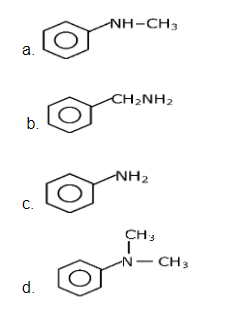Solution:Orange bright dye.

Question 15. What is the correct sequence of reagents used for converting nitrobenzene into m- dibromobenzene?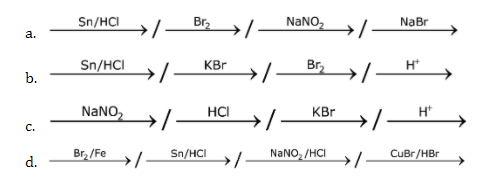Solution: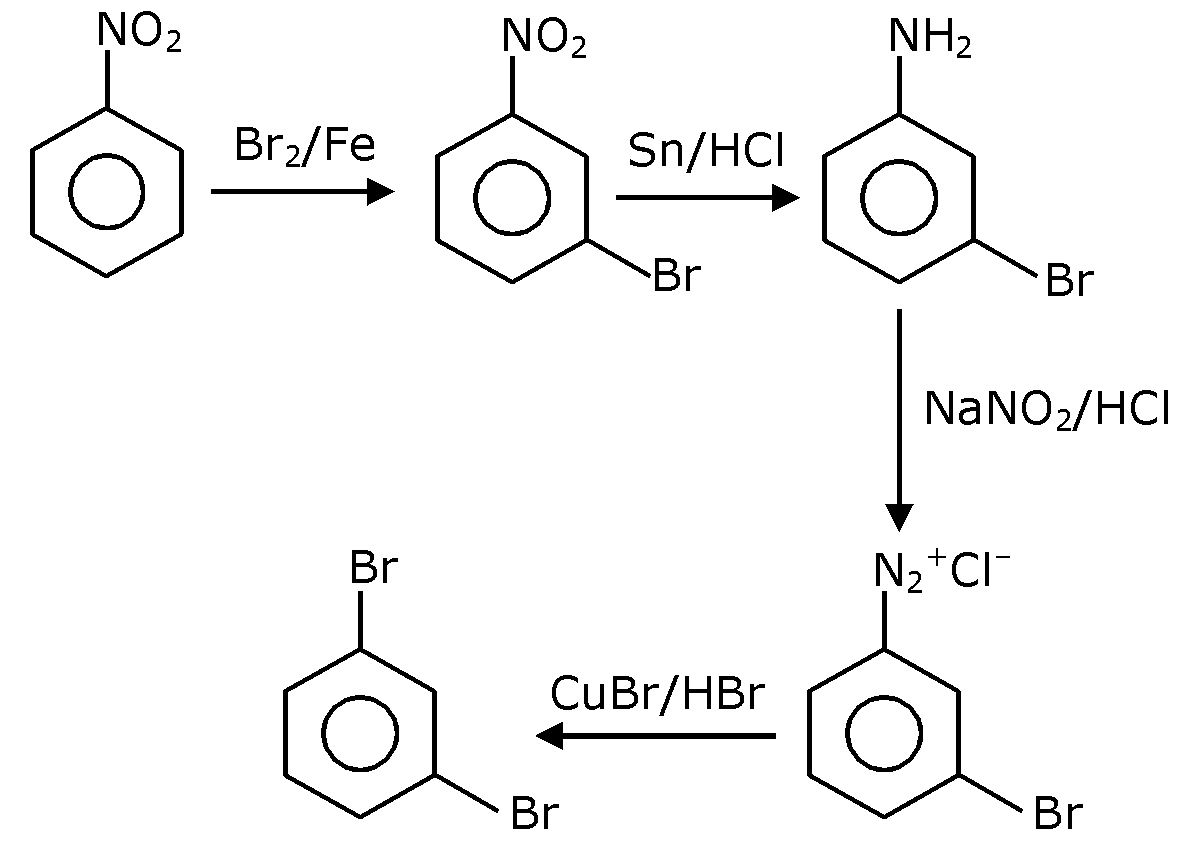Question 16. The correct shape and I-I-I bond angles respectively in I-3 ion are:

1. a. Trigonal planar; 120o
2. b. Distorted trigonal planar; 135o and 90o
3. c. Linear; 180º
4. d. T-shaped; 180º and 90º

Solution:

I-3 has sp3d hybridization (2 BP + 3 LP) and linear geometry.

Question 17. What is the correct order of the following elements with respect to their density?

1. a. Cr < Fe < Co < Cu < Zn
2. b. Cr < Zn < Co < Cu < Fe
3. c. Zn < Cu < Co < Fe < Cr
4. d. Zn < Cr < Fe < Co < Cu

Solution:

Fact Based

Density depends on many factors like atomic mass. atomic radius and packing efficiency.

Question 18. Match List-I and List-II.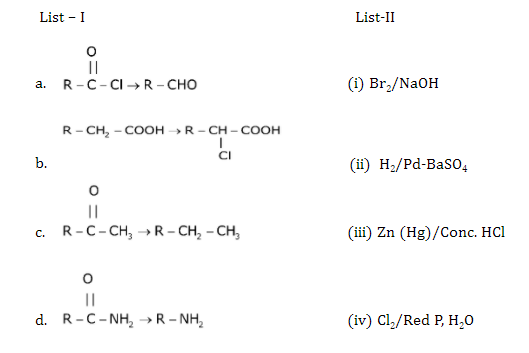1. a. (a)-(ii), (b)-(i), (c)-(iv), (d)-(iii)
2. b. (a)-(iii), (b)-(iv), (c)-(i), (d)-(ii)
3. c. (a)-(iii), (b)-(i), (c)-(iv), (d)-(ii)
4. d. (a)-(ii), (b)-(iv), (c)-(i), (d)-(iii)

Solution:Question 19. In polymer Buna-S: 'S' stands for

1. a. Styrene
2. b. Sulphur
3. c. Strength
4. d. Sulphonation

Solution:

Buna-S is the co-polymer of buta-1,3-diene & styrene

Question 20. Most suitable salt which can be used for efficient clotting of blood will be:

1. a. Mg(HCO3)2
2. b. FeSO4
3. c. NaHCO3
4. d. FeCl3

Solution:

Blood is a negative sol, according to Hardy-Schulz's rule, the cation with high charge has high coagulation power. Hence, FeCl3 can be used for clotting blood.

Section B

Question 1. The magnitude of the change in oxidising power of the MnO4-/ Mn2+ couple is x × 10–4 V, if the H+ concentration is decreased from 1M to 10–4 M at 25°C. (Assume concentration of MnO4- and Mn2+ to be same on change in H+ concentration). The value of x is _____. (Rounded off to the nearest integer).

[Given: 230RT / F = 0.059]

Solution:

5e- + MnO-4 + 8H → Mn+2 + 4H2O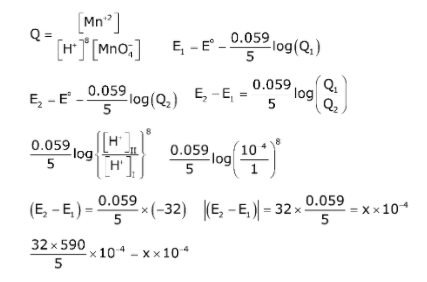= 3776 × 10–4

So, x = 3776

Question 2. Among the following allotropic forms of sulphur, the number of allotropic forms, which will show paramagnetism is ______.

(a) α-sulphur (b) β-sulphur (c) S2-form

Solution:

S2 is like O2 i.e. paramagnetic as per molecular orbital theory.

Question 3. C6H6 freezes at 5.5ºC. The temperature at which a solution of 10 g of C4H10 in 200 g of C6H6 freeze is ________ C. (The molal freezing point depression constant of C6H6 is 5.12C/m).

Solution:

ΔTf = i × Kf × m

= 1 × 5.12 × 10 / 58200 × 1000

∆Tf = 5.12 × 50 / 58 = 4.414

Tf(soution) = Tk(solvent) - ΔT = 5.5 - 4.414 = 1.086oC

≈ 1.09°C = 1 (nearest integer)

Question 4. The volume occupied by 4.75 g of acetylene gas at 50°C and 740 mmHg pressure is _______L. (Rounded off to the nearest integer)

(Given R = 0.0826 L atm K–1 mol–1)

Solution:

T = 50C = 323.15 K

P = 740 mm of Hg = 740 / 760 atm

V = ?

moles (n) = 4.75 / 26 atm

V = 4.75 / 26 × 0.0821 × 323.15 / 740 × 760

V = 4.97 5 Lit

Question 5. The solubility product of PbI2 is 8.0 × 10–9. The solubility of lead iodide in 0.1 molar solution of lead nitrate is x × 10–6 mol/L. The value of x is ________ (Rounded off to the nearest integer)

Given: √2 = 1.41

Solution:

PbI2(s) ⇌ Pb2+ (aq) + 2I- (aq)

S+0.1 2s

KSP (PbI2) = 8 × 109

KSP = [Pb+2][I-]2

8 × 10–9 = (S + 0.1) (2S)2 ⇒ (8 × 10–9 + 0.1) × 4S2

⇒ S2 = 2 × 10–8

S = 1.414 × 10–4 mol/Lit

= x × 10–6 mol/Lit

∴ x = 141.4 141

Question 6. The total number of amines among the following which can be synthesized by Gabriel synthesis is _______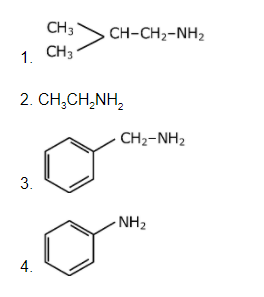Solution:

Only 1o amines can be prepared by Gabriel synthesis.

Question 7. 1.86 g of aniline completely reacts to form acetanilide. 10% of the product is lost during purification. Amount of acetanilide obtained after purification (in g) is ____× 10–2.

Solution:93 g Aniline produce 135 g acetanilide

1.86 g produce 135 × 1.86 / 93 = 2.70 g

At 10% loss, 90% product will be formed after purification.

Question 8. The formula of a gaseous hydrocarbon which requires 6 times of its own volume of O2 for complete oxidation and produces 4 times its own volume of CO2 is CxHy. The value of y is

Solution:

CxHy + 6O2 4CO2 + y/2 H2O

Applying POAC on 'O' atoms

6 × 2 = 4 × 2 + y/2 × 1

y/2 = 4 ⇒ y = 8

Question 9. Sucrose hydrolysis in acid solution into glucose and fructose following first order rate law with a half-life of 3.33 h at 25ºC. After 9h, the fraction of sucrose remaining is f. The value of is _________× 10–2 (Rounded off to the nearest integer)

[Assume: ln10 = 2.303, ln2 = 0.693]

Solution:

$$Sucrose \overset{Hydrolysis}{\rightarrow} Glucose + Fructose$$

t1/2 = 3.33h = 10 / 3h

$$C_{t} = \frac{C_{o}}{2^{\frac{T}{t_{\frac{1}{2}}}}}$$

Fraction of sucrose remaining = f =

$$\frac{C_{t}}{C_{o}} = \frac{1}{\frac{t}{2\frac{T1}{2}}}$$

$$\frac{1}{f} = 2^{t/t_{1/2}}$$

Log (1/f) =

$$log(2^{t/t_{1/2}})$$
= t / t1/2 log (2)

$$\frac{9}{\frac{10}{3}}\times 0.3 - \frac{8.1}{10} - 0.81$$

= x × 10–2

x = 81

Question 10. Assuming ideal behavior, the magnitude of log K for the following reaction at 25ºC is x × 10–1. The value of x is __________. (Integer Answer)

3HC ≡ CH(g) ⇌ C6H6 (l)

[Given: ΔfG° (HC = CH) = – 2.04 × 105] mol–1; ΔfG°(C6H6) = – 1.24 × 105 J mol–1;

R = 8.314 J K–1 mol–1

Solution:

ΔG°r = ΔG°f [C6H6 (l)] - 3 × ΔG°f [HC = CH]

### JEE Main 2021 Chemistry Paper February 24 Shift 2